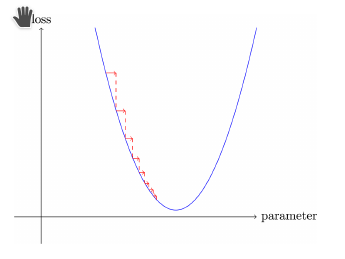# Need clarification about sigmoid function

Can someone explain what does this sentence means ?
" It’s also a smooth curve that only goes up, which makes
it easier for SGD to find meaningful gradients. " – fastai book - page 169 - 2nd line

1 Like

Hi Vivekverma1019,

The idea of sigmoid’s “smooth curve” is that in the middle section of the function there is only a monotonically increasing curve. The reason why this is good for SGD finding “meaningful gradients” is that the gradient in that middle section of the curve is steep. So when you take one step in the direction of the negative gradient you are able to update your weight in a “meaningful” amount.

Notice that this is not the case when you reach the outer sections of the sigmoid function. Hence, why ReLU activation function is used more often since its discovery. So long as your input is positive you will have a steep slope which is “meaningful” for the gradient.

2 Likes

Thanks for responding.
I still have a small doubt. It would be helpful to me if u can clarify that too.
Doubt: We calculate gradients on the graph of loss vs parameters [fig. 1]. Then why the steepness of activation function helps?You’re welcome,

This is because your loss is dependent on the prediction of your model which is in turn dependent on the output of each activation function in your layers. So by simply making sure that the activation function has a shape that produces steep gradients we can influence the overall direction taken on the loss vs param graph.

One way to see this is to realize that backpropigation is simply the gradient at each layer times the gradient of the previous layer with respect to loss. What is each layer? An affine transformation (wx + b) followed by activation function (ReLU/Sigmoid/Tanh, etc…).

1 Like

Thanks, I got it now